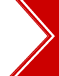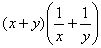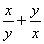Ascent's Courses Business Partnership Jobs @ Ascent Testimonials Success Stories

Data Sufficiency - Quant/Math - CAT 2013

Question 4 the day: August 05, 2003

The question for the day is from the topic of Data Sufficiency.
Mark 1 If the question can be answered by using one of the statements alone, but cannot be answered by using the other statement alone.
Mark 2 If the question can be answered by using either statement alone.
Mark 3 If the question can be answered by using both statements together, but cannot be answered by using either statement alone.
Mark 4 If the question cannot be answered even by using both the statements together.

Is x = y?
A.= 4
B. (x  50)2 = (y  50)2

 (1) 1 (2) 4 (3) 3 (4) 2

Solution:

Statement A.= 4 => 1 + 1 += 4

=>= 2. This is possible when x = y. Hence statement A alone is sufficient.

Statement B. (x  50)2 = (y  50)2 . We cannot say if x = y in this case. Take for example, let x = 100 and y = 0. Then (x  50)2 = (100  50)2 = 502 = 2500.

And (y  50)2 = (0  50)2 = 502 = 2500.

Hence, statement A alone is sufficient and statement B alone is not sufficient.

 Home | Question Bank | Top B-Schools | US B-Schools | GD-PI | Forums | GRE Preparation Chennai | Doubt Fire © 2002 - 10 ASCENT Education all rights reserved.